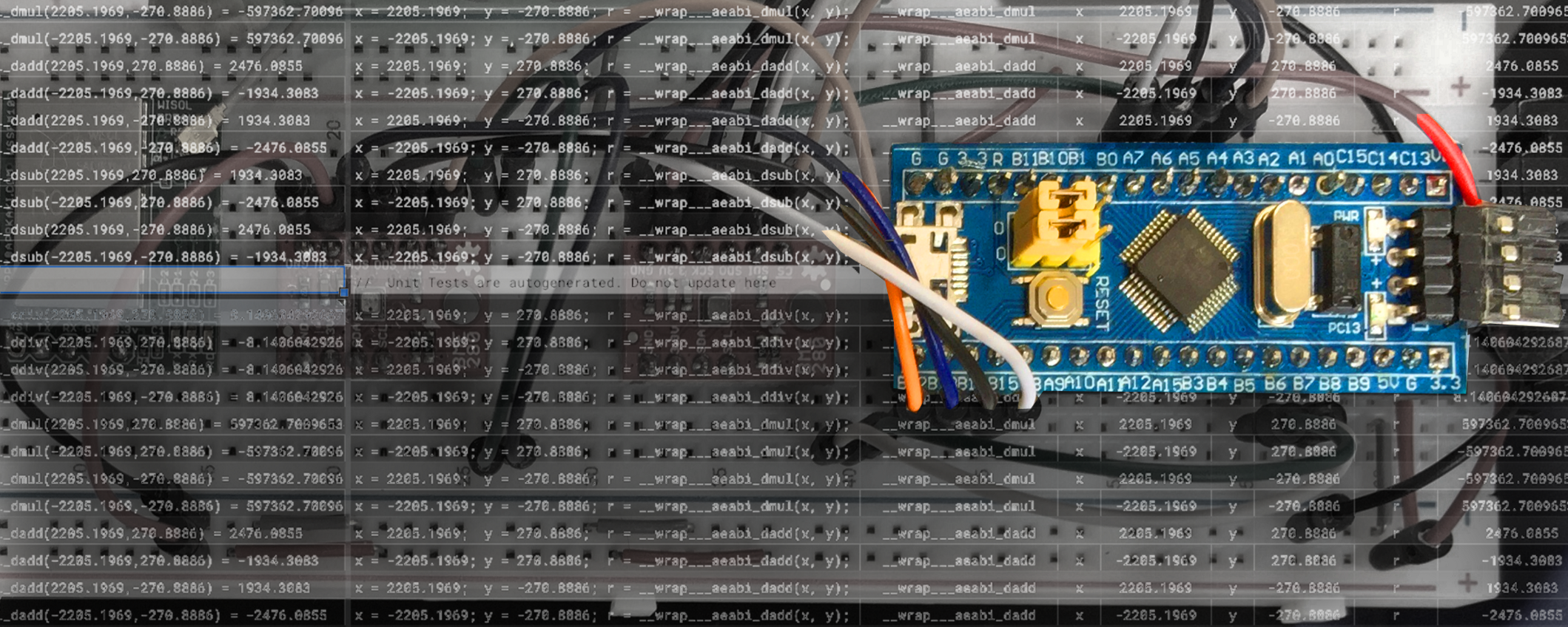# STM32 Blue Pill – Shrink your math libraries with Qfplib

30 Jan 2019 · 9 min read

Check out this magic trick… Why does Blue Pill think that 123 times 456 is 123,456 ???!!! Here’s the full source code.

This article explains how we hacked Blue Pill to create this interesting behaviour, and how we may exploit this hack to cut down the size of our math libraries…

1. What are Math Libraries? How does Blue Pill perform math computations?
2. Qfplib the tiny math library
3. Single vs Double-Precision numbers. Do we really need Double-Precision?
4. Filling in the missing math functions with `nano-float`
5. Testing Qfplib and nano-float
6. My experience with Qfplib and nano-float

This article covers experimental topics that have not been tested in production systems, so be very careful if you decide to use any tips from this article.

# What are Math Libraries? Why so huge?

If you have used math functions like `sin(), log(), floor(), ...` then you would have used the `libm.a` Standard Math Library for C. It’s a bunch of common math functions that we may call from C and C++ programs running on Blue Pill and virtually any microcontroller and any device.

But there’s a catch… The math functions will perform better on some microcontrollers and devices — those that support hardware floating-point operations in their processors.

Unfortunately Blue Pill is not in that list. The math libraries are implemented in software, not hardware. So a single call to `sin()` could actually keep the Blue Pill busy for a (short) while because of the complicated library code. And the complicated math code also causes Blue Pill programs to bloat beyond their ROM limit (64 KB).

Is there a better way to do math on Blue Pill? The secret lies in the Magic Trick…

# Magic Trick Revealed

Remember the above code from the Magic Trick… Why does Blue Pill think that 123 times 456 is 123,456? Let’s look at the Assembly Code (check my previous article for the “Disassemble” task)…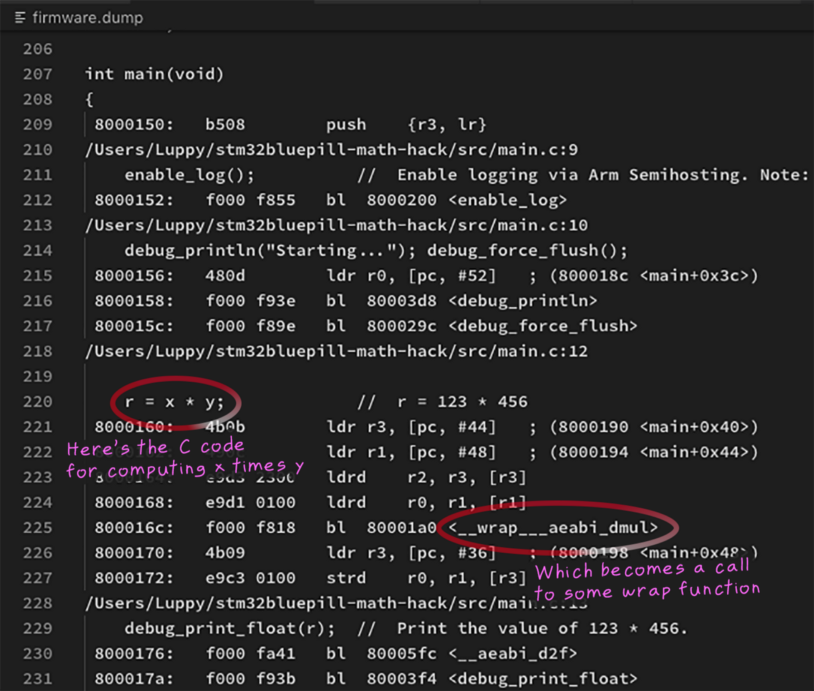Something suspicious is happening here… the line

has actually been compiled into a function call that looks like…

Multiplication of numbers looks simple and harmless when we write it as `x * y`. But remember that `x` and `y` are floating-point numbers. And Blue Pill can’t compute floating-point numbers in hardware. So Blue Pill needs to call a math library function `__wrap___aeabi_dmul` to compute `x * y !`

It looks more sinister now…

Remember this function that appears at the end of the magic trick?

That’s where the magic happens — it returns `123456` for any multiplication of `doubles` in the program. We have intercepted the multiplication operation to return a hacked result.

`__wrap___aeabi_dmul` is typically a function with lots of computation code inside… 1 KB of compiled code. So our code size will bloat significantly once we start using floating-point computation on Blue Pill.

Now what if we do this…

What if we could find a tiny function `qfp_fmul` that multiplies floating-point numbers without bloating the code size? That would be perfect for Blue Pill! `qfp_fmul` actually exists and it’s part of the Qfplib library, coming up next…

💎 About the `wrap`: The `wrap` prefix was inserted because we used this linker option in platformio.ini:` -Wl,-wrap,__aeabi_dmul`. Without `wrap`, the function name is actually `__aeabi_dmul`. By using `wrap` we can easily identify the functions that we have intercepted. Check this doc for more info on AEABI functions.

# Qfplib the tiny floating-point library

Qfplib is a floating-point math library by Dr Mark Owen that’s incredibly small. In a mere 1,224 bytes we get Assembly Code functions (specific to Arm Cortex-M0 and above) that compute…

• `qfp_fadd, qfp_fsub, qfp_fmul, qfp_fdiv_fast`: Floating-point addition, subtraction, multiplication, division
• `qfp_fsin, qfp_fcos, qfp_ftan, qfp_fatan2`: sine, cosine, tangent, inverse tangent
• `qfp_fexp, qfp_fln`: Natural exponential, logarithm
• `qfp_fsqrt_fast, qfp_fcmp`: Square root, comparison of floats

Plus conversion functions for integers, fixed-point and floating-point numbers. What a gem!

With Qfplib we may now intercept any floating-point operation (using the `__wrap___aeabi_dmul` method above) and substitute a smaller, optimised version of the computation code.

Be careful: There are limitations in the Qfplib functions, as described here. And Qfplib only handles single-precision math, not double-precision. More about this…

# Single vs Double-Precision Floating-Point Numbers

You should have seen single-precision (`float`) and double-precision (`double`) numbers in C programs…

Double-precision numbers are more precise in representing floating-point numbers because they have twice the number of bits (64 bits) compared with single-precision numbers (32 bits).

To be more specific… single-precision numbers are stored in the IEEE 754 Single-Precision format which gives us 6 significant decimal digits. So this `float` value is OK because it’s only 6 decimal digits…

But we may lose the seventh digit in this `float` value because 32 bits can’t fully accommodate 7 decimal digits…

And Blue Pill may think that `x` has the same value as `y`. We wouldn’t use `float` to count 10 million dollars (7 decimal digits or more).

With double-precision numbers (stored as IEEE 754 Double-Precision format) we have room for 15 significant decimal digits

Double precision is indeed more precise than single precision. BUT…

1. `doubles` take up twice the storage
2. `doubles` also require more processor time to compute

This causes problems for constrained microcontrollers like Blue Pill. Also if we use common math functions like `sin(), log(), floor()`, … we will actually introduce `doubles` into our code because the common math functions are declared as `double`. (The single-precision equivalent functions are available, but we need to consciously select them as `sinf(), logf(), floorf()`, …)

# Do we really need Double Precision numbers?

I teach IoT. When my students measure ambient temperature with a long string of digits like 28.12345, I’m pretty sure they’re not doing it right.

Sensors are inherently noisy so I won’t trust them to produce such precise double-precision numbers. Instead I would use a Time Series Database to aggregate sensor values (single-precision) over time and compute an aggregated sensor value (say, moving average for the past minute) that’s more resistant to sensor noise.

If you’re doing Scientific Computing, then `doubles` are for you. Then again you probably won’t be using a lower-class microcontroller like Blue Pill. It’s my hunch that most Blue Pill programmers will be perfectly happy with `floats` instead of `doubles` (though I have no proof).

Always exercise caution when using `floats` instead of `doubles`. There’s a possibility that some intermediate computation will overflow the 6-digit limitation of `floats,` like this computation. GPS coordinates (latitude, longitude) may require `doubles` as well.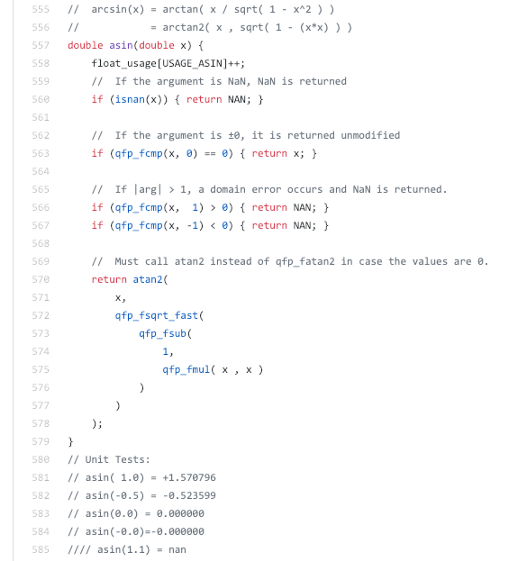# Complete Math Library: nano-floatQfplib provides the basic float-point math functions. Many other math functions are missing: `sinh`, `asinh`, … Can we synthesise the other math functions?

Yes we can! And here’s the proof…

`https://github.com/lupyuen/codal-libopencm3/blob/master/lib/nano-float/src/functions.c`

`nano-float` is a thin layer of code I wrote (not 100% tested) that fills in the remaining math functions by calling Qfplib. `nano-float` is a drop-in replacement for the standard math library, so the function signatures for the math functions are the same… just link your program with `nano-float` instead of the default math library, no recompilation needed.

This looks like a math textbook exercise but let’s derive the missing functions based on Qfplib…

1️⃣ `log2` and `log10` were derived from Qfplib’s `qfp_fln` (natural logarithm function) because…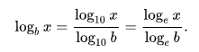2️⃣ `asin` and `acos` (inverse sine / cosine) were derived from Qfplib’s `qfp_fsqrt_fast` (square root) and `qfp_fatan2` (inverse tangent) because…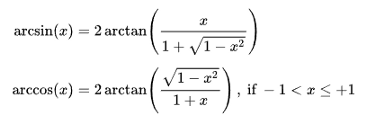3️⃣ `sinh`, `cosh` and `tanh` (hyperbolic sine / cosine / tangent) were derived from Qfplib’s `qfp_fexp` (natural exponential) because…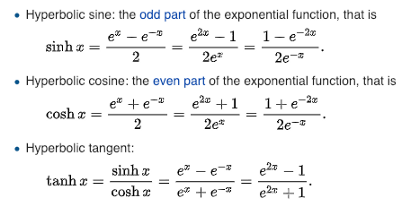4️⃣ `asinh`, `acosh` and `atanh` (inverse hyperbolic sine / cosine / tangent) were derived from `qfplib’s` `qfp_fln` (natural logarithm) and `qfp_fsqrt_fast` (square root) because…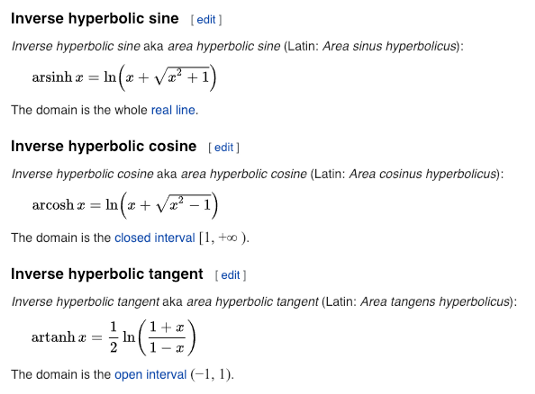So you can see that it’s indeed possible for `nano-float` to fill in the missing math functions by simply calling Qfplib!

But watch out for Boundary Conditions — the code in `nano-float` may not cover all of the special cases like `0, +infinity, -infinity, NaN` (not a number), …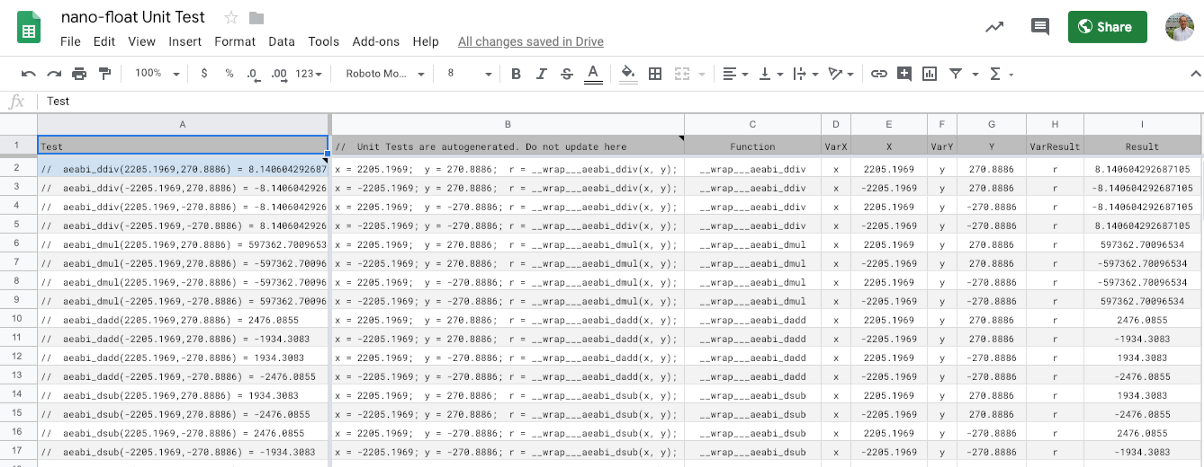# Unit Test with Qemu Blue Pill Emulator

Replacing the standard math library by `nano-float` is an onerous tasks. How can we really be sure that the above formulae were correctly programmed? And that the `nano-float` computation results (single precision) won’t deviate too drastically from the original math functions (double precision)?

It’s not a perfect solution but we have Unit Tests for `nano-float: https://github.com/lupyuen/codal-libopencm3/blob/master/lib/nano-float/test/test.c`

These test cases are meant to be run again and again to verify that the results are identical whenever we make changes to the code. The test cases were automatically extracted from the `nano-float` source code by this Google Sheets spreadsheet…

Unit tests are also meant to be run automatically at every rebuild. But the code requires a Blue Pill to execute. The solution: Use the Qemu Blue Pill Emulator to execute the test cases, by simulating a Blue Pill in software.

So far the test cases confirm that Qfplib is accurate up to 6 decimal digits, when compared with the standard math library (double-precision). Which sounds right because Qfplib uses single-precision math (accurate to 6 decimal digits).

We’ll cover Blue Pill Unit Testing and Blue Pill Emulation in the next article.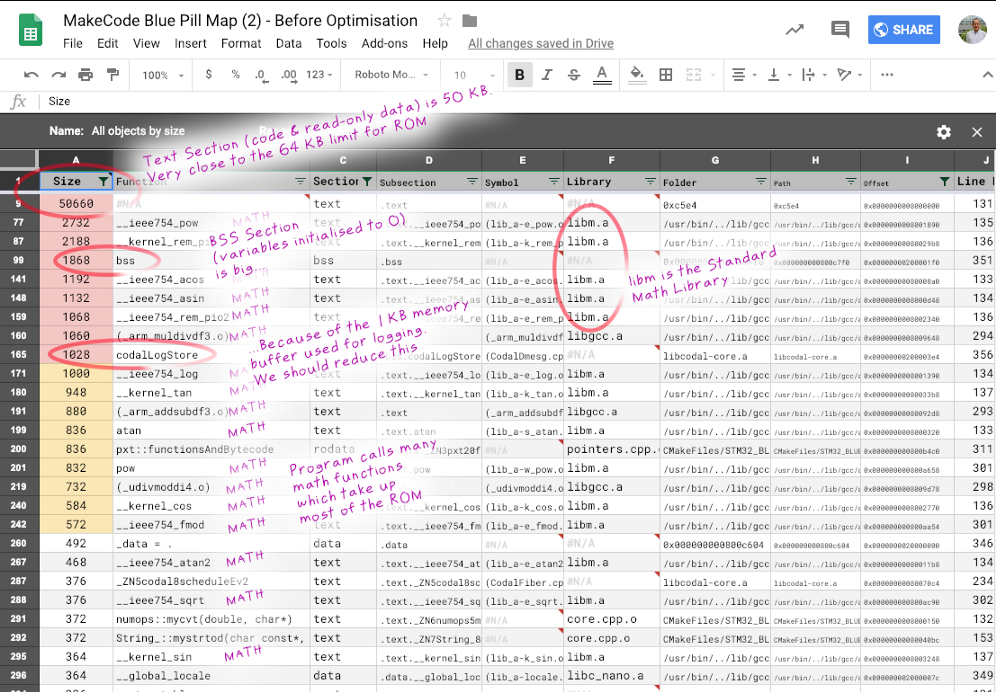# How I used Qfplib and nano-float

MakeCode is visual programming tool for creating embedded programs for microcontrollers (like the BBC micro:bit), simply by dragging and dropping code blocks in a web browser. It’s the perfect way to teach embedded programming to beginners.

While porting MakeCode to Blue Pill, I had trouble squeezing all the code into Blue Pill’s 64 KB ROM. (The BBC micro:bit has 256 KB of ROM, with plenty of room for large libraries.) After compiling for Blue Pill, I noticed that the math libraries were taking huge chunks of ROM storage (roughly 17 KB), which you can see in the spreadsheet above. So I made these changes…

1. Using `__wrap___aeabi_dmul` method described earlier, I intercepted the single-precision and double-precision comparison and arithmetic operations (multiply, divide) and used Qfplib instead. Here’s the complete list of intercepted functions.
2. I used `nano-float` as a drop-in replacement for the standard math library `libm.a`. So all calls to common math functions like `sin(), log(), floor()`, … were handled by `nano-float`.

After optimisation, the ROM usage has dropped drastically, as shown below. So MakeCode might actually run on Blue Pill, assuming we don’t need double-precision math. I haven’t completed my testing of MakeCode on Blue Pill yet — I’m taking a break from the coding and testing to document my MakeCode porting experience, which is what you’re reading now (and more to come).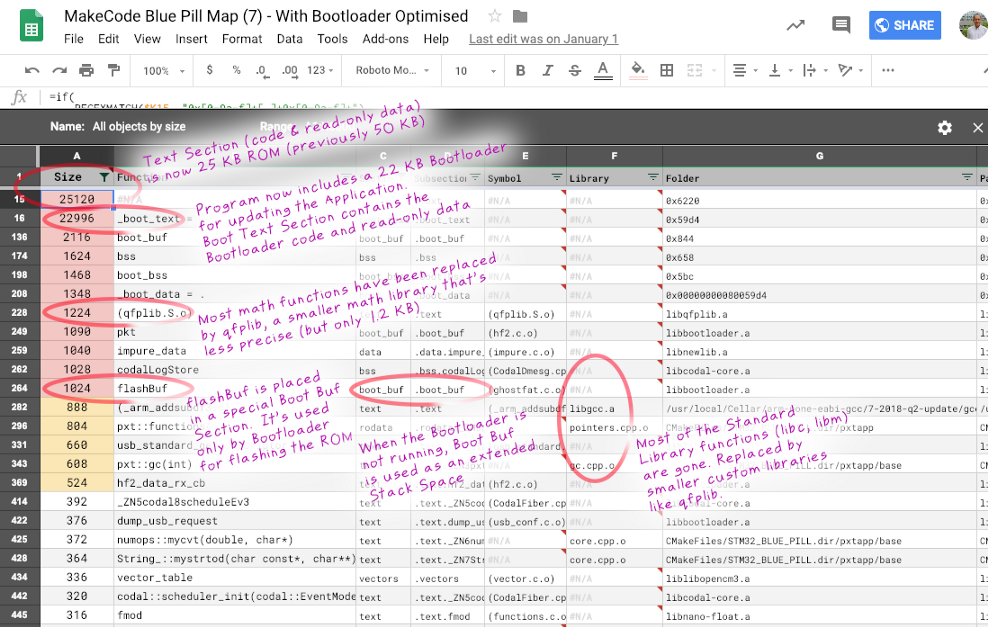# The Daring Proposition

In this article I have argued that we don’t need highly-precise double-precision math libraries all the time. It’s plausible that single-precision math (optimised with Qfplib) is sufficient for IoT and for Blue Pill embedded programs. If we accept this, then the lowly Blue Pill microcontroller will be able to run math programs that were previously too big to fit on Blue Pill. Like the MakeCode visual programming tool.

But this needs to be validated through real-world testing (I’ll be validating shortly through MakeCode). For the daring embedded programmers… All the code you need is already in this article, so go ahead and try it out!

Many thanks to Fabien Petitgrand for the precious feedback